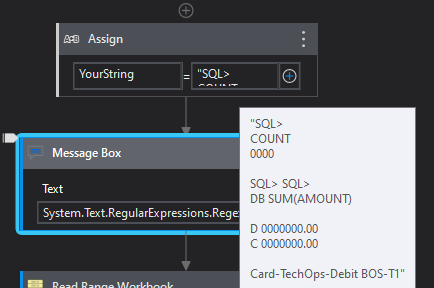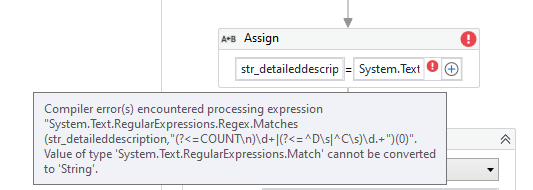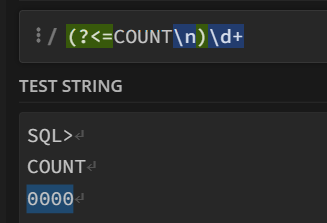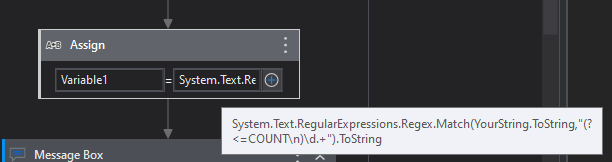# Get specified text from String

Hey All,
I am trying to extract some specified text from below Paragraph.

Input
SQL>
COUNT
0000

SQL> SQL>
DB SUM(AMOUNT)

D 0000000.00
C 0000000.00

Card-TechOps-Debit BOS-T1

Desired Ouput

1. Value of COUNT = 0000
2. Value of D = 0000000.00
3. Value of C = 0000000.00

You can try with this expression

``````System.Text.RegularExpressions.Regex.Matches(YourString,"(?<=COUNT\n)\d+|(?<=^D\s|^C\s)\d.+")(0)

Output -> 0000

System.Text.RegularExpressions.Regex.Matches(YourString,"(?<=COUNT\n)\d+|(?<=^D\s|^C\s)\d.+")(1)

Output -> 0000000.00

System.Text.RegularExpressions.Regex.Matches(YourString,"(?<=COUNT\n)\d+|(?<=^D\s|^C\s)\d.+")(2)

Output -> 0000000.00
``````Regards
Gokul

1 Like

You can also try with this expression @prerna.gupta

``````System.Text.RegularExpressions.Regex.Matches(YourString,"(?<=COUNT\n)\d+|(?<=^D\s|^C\s)\d.+",System.Text.RegularExpressions.RegexOptions.Multiline)
``````Regards
Gokul

Thanks much.
Just wanted to recheck the variable type for Input String ?

This the input variable check out the screenshot @prerna.guptaIn the above screenshot what is data type for YourString ?If data type is string then facing error. Pls refer screenshot

Update the expression

``````System.Text.RegularExpressions.Regex.Match(YourString,"(?<=COUNT\n)\d.+").Tostring

System.Text.RegularExpressions.Regex.Match(YourString,"(?<=D\s)\d.+").Tostring

System.Text.RegularExpressions.Regex.Match(YourString,"(?<=C\s)\d.+").Tostring
``````

Check out the XAML file

RegexMulitple.xaml (5.5 KB)

Regards
Gokul

Checkout this expression for the count

``````System.text.RegularExpressions.Regex.Match(StrVariable,"(?<=COUNT\n)\d+").ToString
``````For values of D and C if those D and C are dynamic try the below
String variable ValueofRows =

``````System.text.RegularExpressions.Regex.Match(StrVariable,"(?s)(?<=AMOUNT\)\n{2}).*(.*?)^\n").ToString
``````

The above will get you those complete rows and will get all the number of rows for eg.

To get all the values

``````System.text.RegularExpressions.Regex.Matches(ValueofRows,"\d+")
``````

if you loop on with the expression above in for each you will get the each and every match so that you can assign them to the datatable or whatever you want.

Hope this Helps

Regards
Sudharsan

Use .ToString at end of the expression @prerna.gupta

With updated expressions also facing same error as above.
I checked the xml file code as well, Can you try to save to output of message box in some variable pls ?
That is what is creating a problem.

Can you tell us the variable type of your input? @prerna.gupta

Check this after giving .tostring in the value field of assign @prerna.gupta

It is String

Try like this @prerna.gupta

``````System.Text.RegularExpressions.Regex.Match(YourString.Tostring,"(?<=COUNT\n)\d.+").Tostring
``````

Check out this screenshotHi @prerna.gupta you can also use this regex

System.Text.RegularExpressions.Regex.Match(“yourString”,“((?<=COUNT\n).\d)|((?<=D\s?).\d)|((?<=C\s?).*\d)”)

With this expression Output is Null

@Tapan_Behera1 ,
With the above expression also its variable type mismatch error.
Can you suggest the datatype in which i can save the output of Regex ?

Have you tried with this expression ? @prerna.gupta

Can you share the exact input here?